Online Tution   »   CBSE Class 10 Science Previous Year...

# CBSE Class 10 Science Previous Year Question Papers with Solutions

The previous year papers of Class 10th Science will help students to understand the pattern and level of questions. CBSE Class 10th Science Previous Year Question Paper with solution will help students to fetch extra marks in science of Class 10th. If students wants to score more than the competition then they have outperform and CBSE Class 10th Science Previous Year Question Paper with solution will help students to pefrom better than competion in their CBSE Class 10th examination.

## Class 10th Science Previous Year Question Paper

On this page, we have given the CBSE Class 10th Science Previous Year Question Paper with solution. The students appearing in CBSE Class 10th Term 2 Exam 2022 must solve the CBSE Class 10th Science Previous Year Question Paper with solution given on this page to pass examination with flying colors.

Also Check:

## Class 10th Science Previous Year Question Paper – 2020

Q. A water submerse rod is used to boil a bucket of water, it required 40 minutes to boil a bucket of water. How to reduce the boiling time of a bucket water?

Answer:- As we know that the main supply voltage cannot be change it will be constant, to reduce the boiling time we should increase the heat produce by the submerse rod, By the formula of power P=V2/R, where supply voltage is constant, we can increase of decrees the resistance of  a rod as resistance is not constant.

Thus R∝l by decreasing the length we can decrease the resistance of the rod so the time required to boil is decrease.

Q. For the given circuit below answer the following questions,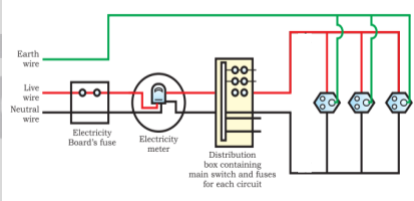• How much supply voltage is required for the following circuit?
• What happens if the earth wire gets damaged in the following circuit?

Answer: (A) The supply voltage required for the following circuit is 220V – 240V.

(B) In this case leakage current does not get grounded due to that the appliances which we used may get damaged.

Q. Which among the following groups belong to Dobereiner triads?

 Group A elements Atomic mass Group B elements Atomic mass Group C elements Atomic mass Co 58.5 Ca 40.1 H 1 Pd 106.4 Zn 65.5 C 12 Pt 195.1 Zr 91.2 Na 23

Ans. Group B and Group C

Explanation: Group B and Group C belongs to the Dobereiner triad as the atomic mass of the middle element is roughly the average of the other two elements.

For Group B

Mass of Zn=Avg of remaining two elements=Sum of two elements2

=40.1+91.22

=65.5

Mass of Zn=65.5

For Group C

Mass of = Avg of remaining two elements=Sum of two elements2

=1+232

=12

Mass of C=12

Now if we calculate for Group A the atomic mass of middle element is not the average of the other two elements.

For Group A

Mass of Pd= Avg of remaining two elements=Sum of two elements2

=58.5+195.12

=126.8

Mass of Pd=126.8

But, Mass of Pd is 106.4 Hence Group A is not Dobereiner triad.

Q. A carbon compound having a melting point of 90 K?

1. a) What is the boiling point of above carbon compound?
2. b) Draw the single covalent bond structure of above carbon compound?

Answer: a) Methane is a carbon compound having a melting point of 90K and boiling point of 111k.

b)The diagram of single covalent bond structure of carbon compound is as bellow,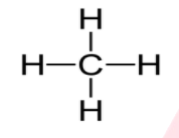Q. Are the characteristics of birds and bats analogous or homogeneous?

Answer: Bats’ wings are folds of skin stretched primarily between elongated fingers. But the wings of the birds are a feather-like covering along the arm. The design of the two wings, their structure and components are therefore very different. They look similar because they share a common use for flying, but their origins are not common. This makes them analogous features.

Q.For the given figure answer the following questions?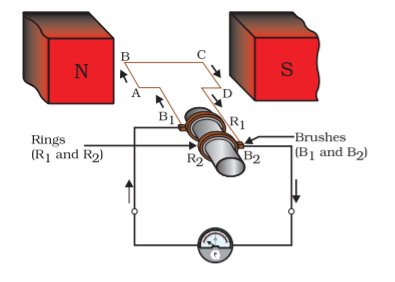• For getting the direct current which type of commutator is used?
• What is the purpose of the galvanometer used in the given arrangement?
• State the law does the given arrangement works? What happens after the half rotation of the motor completes?

1. Split ring type of commutator is used.
2. It is used to show the current flow in the external circuit. It is a device that uses the deflection of a moving coil to measure a small electrical current or a function of the current.
3. Faraday’s law of Electromagnetic Induction. After half a rotation, arm CD begins to move upward, while arm AB begins to move downward. As a result, the directions of the induced currents in both arms change, causing the net induced current to flow in the direction DCBA

Q. Write down the electronics configuration of the following elements,

Tc, Eu, Os, and Hs.

Answer: The electronics configurations of the elements are as follow,

Tc:-[Kr] 4d5 5s2

Atomic number 43,

Valance electron 4d5 5s2

Eu:- [Xe] 4f7 5d1 6s2

Atomic number 63,

Valance electron 4f7 5d1 6s2

Os:-[Xe] 4f145d66s2

Atomic number 76,

Valance electron 4f145d66s2

Hs:-[Rn]7s25f146d6

Atomic number 108,

Valance electron 7s25f146d6

OR

Q. How might you tell the difference between a C2H5OH and a CH3COOH in an experiment? Determine the molecular mass in the above element?

Answer: – Because of its acidic character, CH3COOH turns blue litmus paper red almost instantly, but this does not happen with C2H5OH, which indicates its neutral nature.

When C2H5OH reacts with Sodium metal, it produces hydrogen gas in the form of bubbles, however CH3COOH does not response.

Molecular mass of the element is C2H5OH.

C2H5OH=12×2+1×5+16+1=24+5+16+1=46au

CH3COOH=12+1×3+12+16+16+1=12+3+13+32=44+16=60au

Molecular mass of the element is CH3COOH.

Q. When fertilisation happens, the DNA of both parents mixes, resulting in variety.

Answer: Copying DNA during reproduction is a very important event. Because: The replication of DNA ensures that each daughter cell that is formed at the end of cell division receives the same amount of DNA. If the DNA is not copied, the daughter cells will not receive all the necessary genes that would carry information from one generation to the next generation. There can be a lack of crucial proteins that can be encoded by DNA when DNA cannot be replicated. Copying of DNA can result in recombination leading to evolution. The transfer of genetic material from one generation to another makes the organisms have similar functions. The stability of the population is maintained. When fertilization occurs, the DNA of both parents combines, causing variations to occur.

Q. a)Determine the total resistance of the below gicen circuit?

b) Determine the current flowing in the circuit?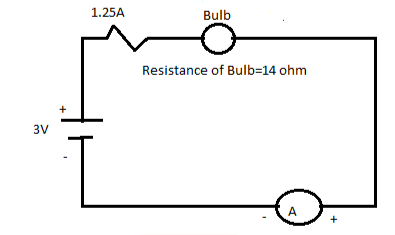The internal resistance of a bulb is 14 ohm

Both the resiistnace are connected in the circuit,

Thus adding the resiistance in series we get,

R=R1+R2

R=14+1.25

R=15.25 ohm

Now to calculate the current flowing in the circuit is I=V/R.

The given voltage is V=3V.

Substitute the value in above given ohms law we get,

I=3/15.25

I= 0.197 A.

Q.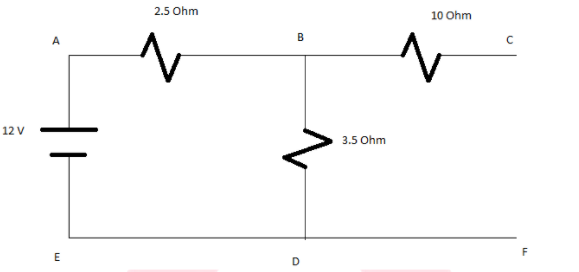1. a) Determine equivalent resistance of the circuit
2. b) Total current flowing in the circuit
3. c) current flowing in the branch BC

Solution:

1.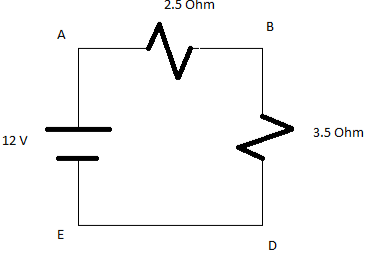As the path C and F are not connected or open. The current will not flow in the open circuit so circuit will reduce as above and no current will flow through resistance 10 Ohms.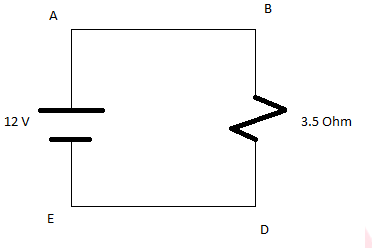Therefore equivalent resistance is equal to Req=6 Ohms

b)Total current flowing in the circuit is I=V/R

I=12/6=2A

c)The current in the branch BC will be zero as the circuit is open. So no current will flow through it.

Q. Find the value of total current in the circuit also determine the value of equivalent resistance in the circuit.

Solution: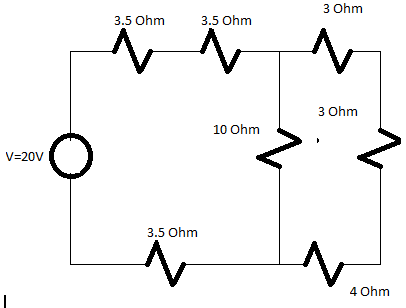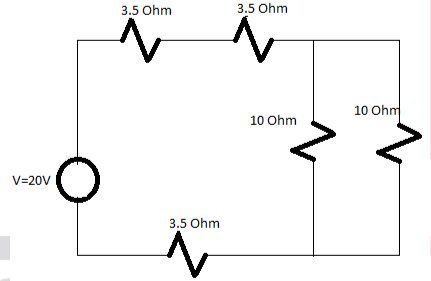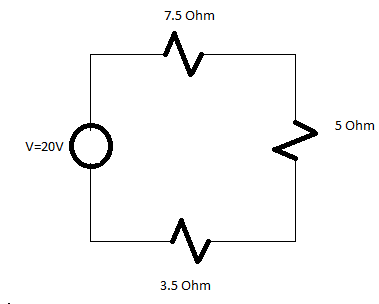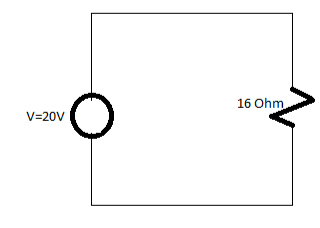Total Resistance=16 Ohm

Total current=V/Total resistance

=20/16

I=1.25A

Q. How might you tell the difference between a C2H5OH and a CH3COOH in an experiment? Determine the molecular mass in the above element?

Answer: Because of its acidic character, CH3COOH turns blue litmus paper red almost instantly, but this does not happen with C2H5OH, which indicates its neutral nature.

When C2H5OH reacts with Sodium metal, it produces hydrogen gas in the form of bubbles, however CH3COOH does not response.

Molecular mass of the element is C2H5OH.

C2H5OH=12×2+1×5+16+1=24+5+16+1=46au

CH3COOH=12+1×3+12+16+16+1=12+3+13+32=44+16=60au

Molecular mass of the element is CH3COOH.

Q. From the given arrangement, answer the following questions?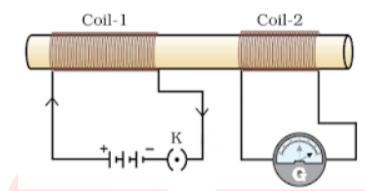• What will happen if we give supply to the coil 1?
• What happens if we change the coil 1?

1. Coil 1 is connected to the battery and the key which is open. Whenever we closed the key, the supply starts flowing through the coil and on another side in coil 2 the galvanometer connected instantly jumps to one side and just as quickly returns to zero, indicating a momentary current.
2. From the given arrangement, we see that the battery and key is connected in the coil 1 and the galvanometer is connected to the coil 2. Whenever there is change in the coil 1 there is a current induced in coil 2.

Q. Explain Mendel’s experiments using a pea plant to demonstrate that characteristics are inherited separately.

Answer: Mendel succeeded in proving that the traits are inherited independently with the help of pea plants. Mendel selected two different pea plants with the following characteristics: Pea shape: Round or wrinkled Pea colour: Yellow or green Flower colour: Purple or white Flower position: Terminal or axial Plant height: Tall or low Pod shape: Bloated or pinched Pod colour: Yellow or green Certain hereditary principles were developed based on Mendel’s experiments: Fundamental Heredity Theory: According to this theory, offspring have inherited their genes from their parents. Principle of Segregation: It has been studied that during reproduction, the factors that determine the traits are separated in reproductive cells and this process is called meiosis and they reunite during fertilization. Principle of independent sorting: There are different genes on different chromosomes that can be inherited independently of each other.

## Class 10th Science Previous Year Question Paper – 2019

Q.  From the figure answer the following question.

• Which are the alkali metals?
• Which of the following has lowest and highest atomic numbers?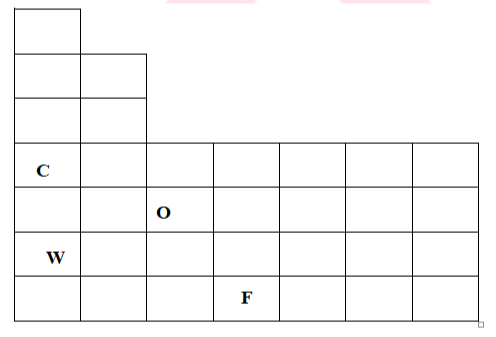Ans. 1. Elements C and W are alkali metals

Explanation: The alkali metals are found in group 1 Lithium, Sodium, Potassium, Rubidium, Caesium and Francium. Hence, C and W are alkali metals.

1. Element C has lowest atomic number and element F has highest atomic number.

Explanation: The element C is present at fourth period of group 1 hence it has lowest atomic number and element F is present at seventh period of group four hence it has highest atomic number. Since atomic number increases as the period and group increases.

Q. which among the following groups belong to Dobereiner triads?

 Group A elements Atomic mass Group B elements Atomic mass Group C elements Atomic mass S 32.1 Be 9.0 Ag 107.9 Co 58.9 Hf 178.5 Au 197.0 Kr 83.8 Bi 209.0 Ta 181.0

Ans. Group A.

Explanation: Group A belongs to the Dobereiner triad as the atomic mass of the middle element is roughly the average of the other two elements.

For Group A

Mass of Co=Avg of remaining two elements=Sum of two elements2

=32.1+83.82

=58.9

Mass of Co=58.9

Now if we calculate for Group B and Group C the atomic mass of middle element is not the average of the other two elements.

For Group B

Mass of Hf= Avg of remaining two elements=Sum of two elements2

=9.0+209.02

=109

Mass of Hf=109

But, Mass of Hf is 178.5 Hence, Group A is not Dobereiner triad.

For Group C

Mass of Au= Avg of remaining two elements=Sum of two elements2

=107.9+1812

=144.5

Mass of Au=144.5

But, Mass of Au is 197.0

Hence, Group A is not Dobereiner triad.

Q. The element given is C12H24 and C12H22.

1. a) Determine the molecular mass in the above element?
2. b) Calculate the difference in the formula of above given element?

Answer: a) The molecular mass of the above element are as bellow,

C12H24=12×12+24×1=144+24=168au

C12H22=12×12+22×1=144+22=166au

1. b) When we look at the formula of C12H24 and C12H22.

C12H24 is an alkenes while C12H22 is an Alkynes both differ by H2 unit.

Q. Name the following structure of different compounds.

1. i)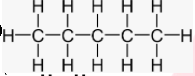2. ii)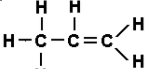3. iii)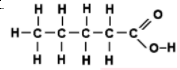i) Pentaneii) Alkenes (Propene)iii) Caroxylicacids (Pentanoic acid)Q5 Students performing the experiment with the setup shown below in their practical lab.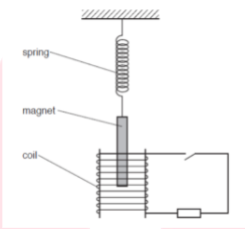• State the principle on which the given setup works?
• What happens if the coil gets damaged?

• The given setup works on the principle of the electromagnetic induction. The generation of an electromotive force throughout an electrical conductor in the presence of a changing magnetic field.
• As we see that, the coil is wounded around a magnet if that coil gets damaged the excessive current flows through the coil is started flowing the whole setup due to the leakage of the current.

Q.6 From the following figure answer the questions?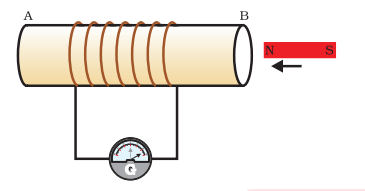• If the magnet is placed near the point B, what do you observe?

Is there any change in the galvanometer which is connected there?

• What happen if you put the magnet away from the point B?

1. As we see that, a coil of wire AB has the higher number of turns and also it is connected to the galvanometer. Whenever the magnet is placed near the point B of the coil, the needle of the galvanometer is moves towards right direction which shows the presence of current in the coil.
2. When you take the magnet away from the coil the deflection of the galvanometer becomes zero. As the galvanometer is device that uses the deflection of a moving coil to measure a small electrical current or a function of the current

Q. Predict the equations for the hydride of the following elements using Mendeleev’s Periodic Table: Na, Cu, B, Ti, and Ca

Answer: Sodium (Na) is a member of Group I. Mendeleev’s periodic table member. As a result, its valency is 1. As a result, the hydride formula is NaH.

Copper (Cu) is a member of Group I. Mendeleev’s periodic table member. As a result, its valency is 1. As a result, the hydride formula is CuH.

Boron (B) is a member of Group III. Mendeleev’s periodic table member. As a result, its valency is 3. As a result, the hydride formula is BH3.

Titanium (Ti) is a member of Group IV. Mendeleev’s periodic table member. As a result, its valency is 4. As a result, the hydride formula is TiH4.

Calcium (Ca) is a member of Group II. Mendeleev’s periodic table member. As a result, its valency is 1. As a result, the hydride formula is CaH2.

Q2) If we cook food in a gas/kerosene stove and after cooking we seen that the base of pan is getting black.

1. a) What does the black base of pan indicates?
2. b) If the flame is sooty what does it indicates?

1. If we cook food in a gas/kerosene stove and after cooking we seen that the base of pan is getting black it indicates that the air holes are blocked and the fuel is getting wasted while cooking food.
2. When we burn saturated hydrocarbons it’s give a clean flame but if we limit the supply of air the proper combustion of fuel will not takes place so it gives sooty flame.

Q3 A chemical substances containing carbon with a boiling point of 162.15 °C.

a)What is the melting point of the above element?

b) What is the structure of the solitary covalent bond in the above element?

Answer: a) Methane is a chemical substances containing carbon having a boiling point of 90K or 162.15 °C and melting point of 90k or -183.15°C.

The dot structure of methane is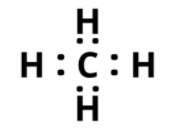b)The diagram of single covalent bond structure of carbon compound is as bellow,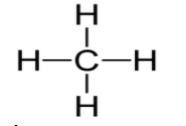Q.

1. Determine the total resistance in the given circuit.
2. Find the value of current flowing in the circuit.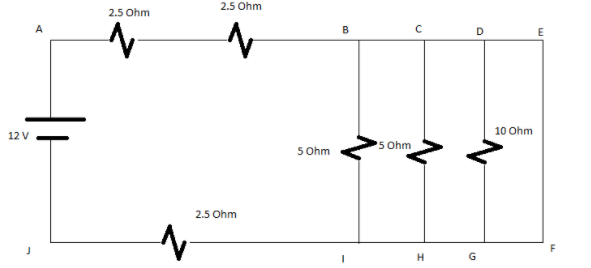Solution: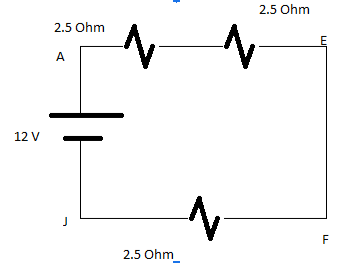As we know that the current will flow from the path where the resistance will be less so the current will not flow through 5 Ohm and 10 Ohm branches.

So circuit can drawn as above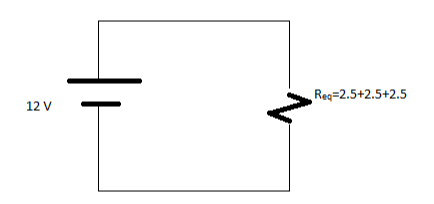Req=7.5 Ohm

The value of current flowing through circuit is I=V/R

I=12/7.5

I=1.6 A

Q5) Calculate the total value of Resistance in the below circuit also determine the total value of current?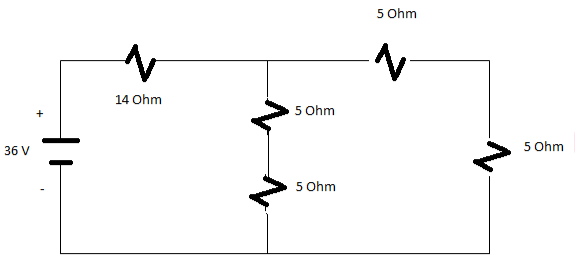Solution: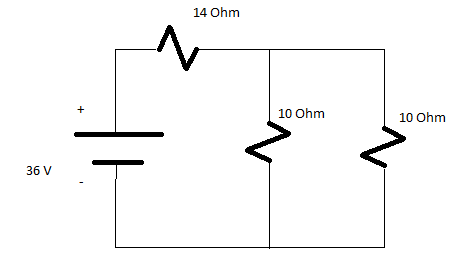Solving parallel branches 10 Ohm and 10 Ohm

(1/10)+(1/10)=5 Ohm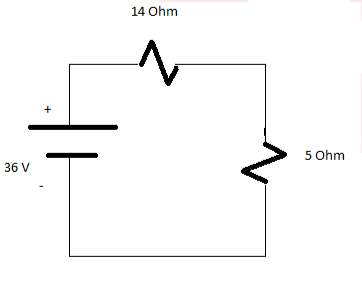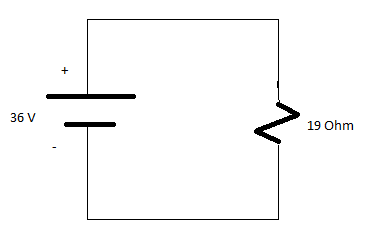Req=19 Ohms

Total Current=V/Req=36/19

I=1.895 A

Q6 Why are people belonging to the same species so different in size, colour and appearance in appearance?

We all come from Africa, where we have lived for the last few thousand years. The earliest members of the human species, Homo sapiens, can be traced there. Our genetic footprints can be traced back to our African roots. A few hundred thousand years ago, some of our ancestors left Africa while others stayed. As residents spread across Africa, migrants slowly spread across the planet, from Africa to West Asia, then to Central Asia, Eurasia, South Asia, and East Asia. They travelled to Australia via the islands of Indonesia and the Philippines and crossed the Bering land bridge to America. They didn’t walk in a single line, so obviously they didn’t travel for the sake of travelling. They walked back and forth, with groups sometimes separating, sometimes coming back to mingle, even moving in and out of Africa.

Like all other species on the planet, they had arisen as an accident of evolution, trying to live their lives as best as possible.

Q1 Akash is sitting in a classroom with one of your backs to one of the walls. A strong field of magnet on your right side a laser beam is deflects which moves from the back wall to the front wall horizontally. What will be the magnetic field’s direction?

• Fleming’s left-hand rule can be used to determine the magnetic field’s direction. The magnetic field on the inside of the chamber will be perpendicular to the direction of current and the direction of deflection/force, which may be either upward or downward.
• As the negatively charged electrons go from the back wall to the front wall, the current flows from the front wall to the back wall. In addition, the magnetic force is directed rightward.
• Using Fleming’s left-hand rule, it can now be determined that the magnetic field inside the chamber is pointing downward.

Or

What is the difference between a solenoid and a magnet?

Answer: A long coil of insulated copper wire circular loops is known as a solenoid. Magnetic field lines from around the solenoid when electricity runs through it. It produces a magnetic field that is very comparable to that of a bar magnet.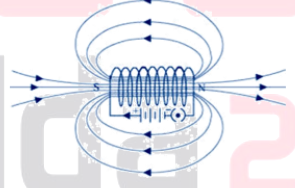The solenoid repels the bar magnet when the North Pole is brought close to the end connected to the battery’s negative contact.

We can conclude that they are like poles because they repel, i.e., the end attached to the -ve end is the North Pole. As a result, the south pole of the solenoid would be the positive end.

Q2 Explain Mendel’s experiments using a pea plant to demonstrate that characteristics are inherited separately.

Mendel succeeded in proving that the traits are inherited independently with the help of pea plants. Mendel selected two different pea plants with the following characteristics: Pea shape: Round or wrinkled Pea colour: Yellow or green Flower colour: Purple or white Flower position: Terminal or axial Plant height: Tall or low Pod shape: Bloated or pinched Pod colour: Yellow or green Certain hereditary principles were developed based on Mendel’s experiments: Fundamental Heredity Theory: According to this theory, offspring have inherited their genes from their parents. Principle of Segregation: It has been studied that during reproduction, the factors that determine the traits are separated in reproductive cells and this process is called meiosis and they reunite during fertilization. Principle of independent sorting: There are different genes on different chromosomes that can be inherited independently of each other.

Or

Draw the covalent bond structure of the bellow elements?

a)C3H8

b)CH3COOH

c)CH3CH2OH

d)Ketone

a) C3H8:-Propane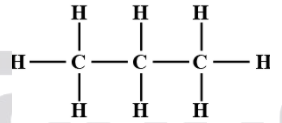b) CH3COOH:-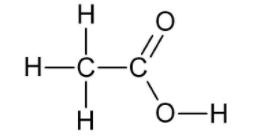CH3CH2OH:- Alcohol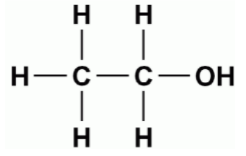d)Ketone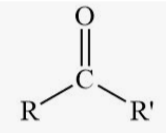## Class 10th Science Previous Year Question Paper: FAQs

Q. When will CBSE conduct the Class 10th Term 2 Examination?

CBSE will begin Class 10th Term 2 Examination from 26th April 2022 onwards.

Q. Where I can get CBSE Class 10th Science Previous Year Question Papers?

On this page, we have given the CBSE Class 10th Science Previous Year Question Papers. For other subjects, you can visit the official website of Adda247 School.

Q. How can I get full marks in science class 10?
To get full marks in science in class 10th the students have to outperform. The students have to complete all the questions within the time and must solve the CBSE Class 10th Science Previous Year Question Papers given on this page.

Q. Which website is best for previous year’s question papers of CBSE Class 10th?

The official website of Adda247 school is best to get all the previous year’s question papers for CBSE Class 10th.

Sharing is caring!

Thank You, Your details have been submitted we will get back to you.